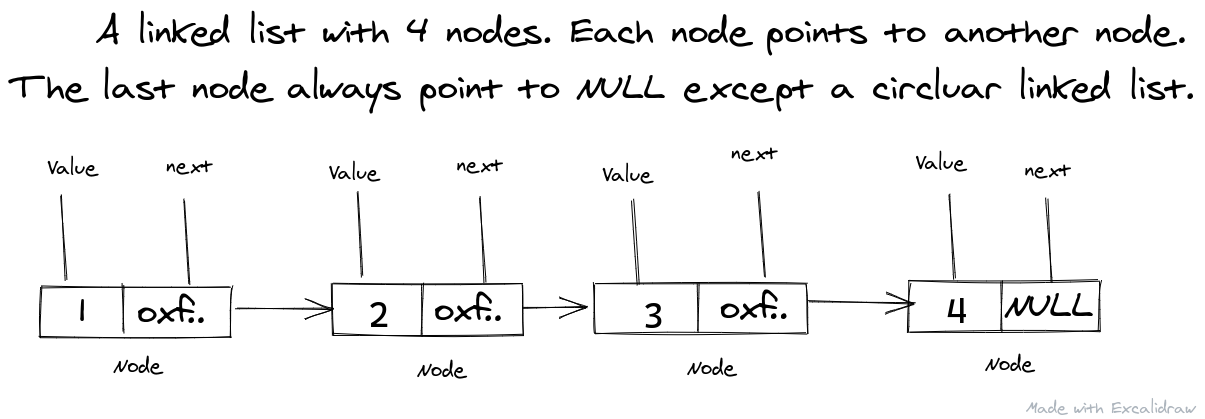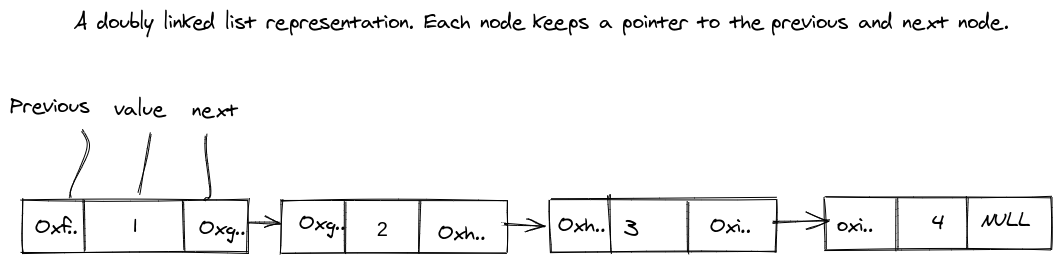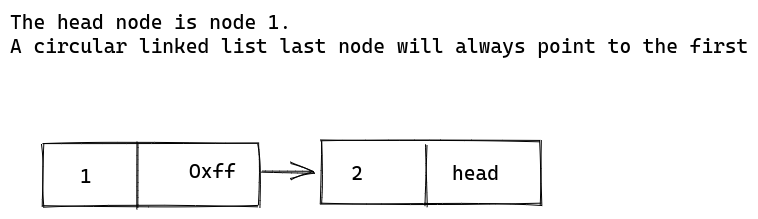Linked list was the first data structure that gave me tough time. I wanted to understand deeply the concept of storing next node pointers - memories, linking etc. I had so much help from Twitter and the course material I was using. I now have a very good understanding of linked lists, this doesn't mean I can answer every linked list question perfectly however, I have solved at least 80-90% of the linked list question on LeetCode.

## What is a Linked List?

Of course, lists that are linked together. The first time I heard of this, I thought linked lists were contiguous arrays like `[1,2,3,4][5,6,7]`. Haha! I wasn't far from the meaning, just that it wasn't as I thought.

A linked list is a continuous list of nodes where a node is a block structure housing the node value and a pointer (or memory) address to the next node. Each node from the head node has a next pointer that keeps the address of the next till it gets to the last node that points to nothing.

The connection from node to node differentiates it from the normal list or array. Arrays don't keep track of their next values or other values unlike the linked lists.

Here is a representation of a linked list:In summary, a linked list is a collection of nodes pointing to each other in no manner, actually.

There are currently three types of linked list:

The above are the basic three types, other sub derivations can be made e.g circular doubly linked list. The image above is an example of a singly linked list.

A singly linked list is defined by its node. A singly linked list node has a value and the next pointer, the linked list diagram below is an example of a singly linked list.A singly linked list node implementation is like this:

Node.py
``````class Node:
def __init__(self, value):
self.value = value
self.next = None  ``````

In JavaScript:

Node.js
``````class Node {
constructor(value) {
this.value = value
this.next = null
}
}``````

A linked list can be easily defined as:

In Python
``````llist = Node(10)
llist.next = Node(11)``````
In JavaScript
``````let llist = new Node(10)
llist.next = new Node(11)``````

The stress of instantiating a new node class everytime pushses us to write a class for the Linked List itself. It isn't necessary but advised, I'll talk on the linked list class itself in subsequent articles.

In simple words, this linked list node is linked at both end. A doubly linked list node points to the previous node and the next node.

Here is a graphical representation:The implementation of a doubly linked list node is:

Node.py
``````class Node:
def __init__(self, value):
self.value = value
self.previous = None
self.next = None``````
Node.js
``````class Node {
constructor(value) {
this.value = value
this.previous = null
this.next = null
}
}``````

It's easy just like the singly linked list.

The circular linked list node is a singly linked list except that the last node always point to the first node.

Here's a diagram:The implementation is the same as the singly linked list except that the linked list class itself ensures that every new node sets its next pointer to the head of the list.

That's all for circular.

## Conclusion

In this article, I discussed the nodes for the different type of lists. Yes, I didn't create or describe the linked list class itself, that will be treated in the next article.

A linked list is basically a collection of nodes keeping pointers to their next nodes ( singly ), previous and next ( dobuly ) and a pointer to the end ( circular ).

If you happen to read this, do comment. I'm always up for corrections and compliments :)